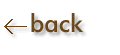Powers of Ten:  Addition and Subtraction

Exponents must be the same of the operation cannot be performed.  If the exponents do not agree, change the decimal and power of ten notation so as to agree with the larger number.

Keep the same power of ten.

Examples:

4.2 x 103     =    4.2 x 103

+    2   x 103    =    0.02 x 103

_____________________________

(CANNOT DO)    4.22 x 103    =    4.2 x 103

(Use correct scientific notation.  Use correct number of significant figures.)